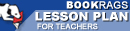Lesson Plans

# Journey Through Genius: The Great Theorems of Mathematics Quiz | Eight Week Quiz A

This set of Lesson Plans consists of approximately 142 pages of tests, essay questions, lessons, and other teaching materials.
 View a FREE sampleName: _________________________ Period: ___________________

This quiz consists of 5 multiple choice and 5 short answer questions through Euclid's Proof of the Pythagorean Theorem.

## Multiple Choice Questions

1. How did Lindeman prove his conclusion?
(a) Lindeman proved that some numbers are constructable without the use of a compass.
(b) Lindeman proved that square roots are irrational numbers.
(c) Lindeman proved that all numbers are constructable with a compass and ruler.
(d) Lindeman proved that some numbers are not constructable with only a compass and straight-edge.

2. Who was the author of the book Elements?
(a) Einstein.
(b) Lindemann.
(c) Hippocrates.
(d) Euclid.

3. What was true about Hippocrates's proof?
(a) It was useful for circles.
(b) The proof was exceedingly difficult and not understood at the time.
(c) It was fairly easy and simple.
(d) The proof was easy if their was advanced technology available.

4. Which of the following was NOT one of the things Dunham claimed was ingenious about Euclid's proof of the Pythagorean theorem?
(a) Euclid used his own axioms and propositions to show relationships,
(b) Euclid constructed squares on the sides of right triangles.
(c) Euclid used propositions about similar angles and parallel lines.
(d) Euclid stated that the diagonal hypotenuse of a right triangle is equal to the sums of the squares of the two legs.

5. Which words best describe how solid proofs were developed in Elements?
(a) Programmed order.
(b) Axiomatic framework.
(c) Simple arguments.
(d) Inverted scaffold.

## Short Answer Questions

1. What did Euclid do in his 48th proposition?

2. That properties of specific shapes were early Egyptians aware of?

3. What was Hippocrates famous for?

4. Which of the following is an example of a postulate that must be accepted in Elements?

5. Which of the following was NOT one of Gauss' discoveries?

(see the answer key)

 This section contains 404 words (approx. 2 pages at 300 words per page) View a FREE sampleCopyrightsJourney Through Genius: The Great Theorems of Mathematics from BookRags. (c)2019 BookRags, Inc. All rights reserved.
Follow Us on Facebook